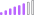cancel
Showing results for
Did you mean:Post Patron

## Relative Date Slicer AND Date Range

Hello Community -

I am trying to figure out a way to be able to use two types of date slicers in the same report.

So I have a report that I use the Relative Date slicing on most of the time, but on occasion I want to be able to select a specific range rather than the last 1 week or 1 month.

Is there an easy way to toggle between the 2 types of date filters on the fly?

I currently and trying to just use a button and two bookmarks to change between the two slicer types, but I cannot get the filters to clear when I go back and forth.  By that I mean, if I select in the last 1 week from the relative filter, and then change to the range filter, the relative filter remains for the last 1 week and then I cannot really look at  date range outside of that.

I would guess there is a simple fix to the solution I am attempting above, but I cannot seem to figure it out.

Any help in any way would be appreciated!

Thanks

Ryan F

1 ACCEPTED SOLUTIONCommunity Support

Using bookmark is correct, you only need to disable the sync slicers between different pages. For more details, please refer to doc below:

https://docs.microsoft.com/en-us/power-bi/visuals/power-bi-visualization-slicers#sync-and-use-slicer...

Community Support Team _ Jimmy Tao

If this post helps, then please consider Accept it as the solution to help the other members find it more quickly.

6 REPLIES 6Anonymous
Not applicable

Yeah I am struggling to acheive this. I have it working if your page only has a single level of bookmarks. However I now have a expanding filter pane that opens or closes, so having another level of bookmarks under that duplicated just to allow flexability of using a date filter properly is annoying. I notice other filters, you can create two off the same field and it allows you to choose different type of display, like one a list, one a dropdown, but if you try this with a date it causes both slicers to change to the same type. Also by using bookmark the filters dont sync so you can't just hide one and display the other as both will be in effect on the visulisation, so your data is wrong. Very annoying and yet to find a decent solution to this problem.New Member

Hi Ryan,

If possible could you help me how you develop the solution in your case as i also need to develop the same kind of soluyion in my report.Super User

You have to use Time intelligence for that. It can take date from slicer or end date of the range and work on that.

For week refer this file:https://www.dropbox.com/s/d9898a48e76wmvl/sales_analytics_weekWise.pbix?dl=0

Example of time intelligence. USe with a date calendar

``````MTD Sales = CALCULATE(SUM(Sales[Sales Amount]),DATESMTD('Date'[Date]))
last MTD Sales = CALCULATE(SUM(Sales[Sales Amount]),DATESMTD(dateadd('Date'[Date],-1,MONTH)))
last MTD (complete) Sales =  CALCULATE(SUM(Sales[Sales Amount]),DATESMTD(ENDOFMONTH(dateadd('Date'[Date],-1,MONTH))))
last year MTD Sales = CALCULATE(SUM(Sales[Sales Amount]),DATESMTD(dateadd('Date'[Date],-12,MONTH)))
last year MTD (complete) Sales =  CALCULATE(SUM(Sales[Sales Amount]),DATESMTD(ENDOFMONTH(dateadd('Date'[Date],-12,MONTH))))

last QTR same Month (complete) Sales =  CALCULATE(SUM(Sales[Sales Amount]),DATESMTD(ENDOFMONTH(dateadd('Date'[Date],-1,Qtr))))

MTD (Year End) Sales =  CALCULATE(SUM(Sales[Sales Amount]),DATESMTD(ENDOFYEAR('Date'[Date])))
MTD (Last Year End) Sales =  CALCULATE(SUM(Sales[Sales Amount]),DATESMTD(ENDOFYEAR(dateadd('Date'[Date],-12,MONTH),"8/31")))

QTD Sales = CALCULATE(SUM(Sales[Sales Amount]),DATESQTD(('Date'[Date])))

Last QTD Sales = CALCULATE(SUM(Sales[Sales Amount]),DATESQTD(dateadd('Date'[Date],-1,QUARTER)))
Last complete QTD Sales = CALCULATE(SUM(Sales[Sales Amount]),DATESQTD( ENDOFQUARTER(dateadd('Date'[Date],-1,QUARTER))))

Last to last QTD Sales = CALCULATE(SUM(Sales[Sales Amount]),DATESQTD(dateadd('Date'[Date],-2,QUARTER)))
Next QTD Sales = CALCULATE(SUM(Sales[Sales Amount]),DATESQTD(dateadd('Date'[Date],1,QUARTER)))

Last year same QTD Sales = CALCULATE(SUM(Sales[Sales Amount]),DATESQTD(dateadd('Date'[Date],-1,Year)))
Last year same QTD Sales = CALCULATE(SUM(Sales[Sales Amount]),DATESQTD(ENDOFQUARTER(dateadd('Date'[Date],-1,Year))))

trailing  4 QTR = CALCULATE(SUM(Sales[Sales Amount]),dateadd('Date'[Date],-4,QUARTER))

YTD Sales = CALCULATE(SUM(Sales[Sales Amount]),DATESYTD(('Date'[Date]),"12/31"))
This Sales = CALCULATE(SUM(Sales[Sales Amount]),DATESYTD((ENDOFYEAR('Date'[Date])),"12/31"))

Last YTD Sales = CALCULATE(SUM(Sales[Sales Amount]),DATESYTD(dateadd('Date'[Date],-1,Year),"12/31"))
Last YTD complete Sales = CALCULATE(SUM(Sales[Sales Amount]),DATESYTD(ENDOFYEAR(dateadd('Date'[Date],-1,Year)),"12/31"))
Last to last YTD Sales = CALCULATE(SUM(Sales[Sales Amount]),DATESYTD(dateadd('Date'[Date],-2,Year),"12/31"))

Year behind Sales = CALCULATE(SUM(Sales[Sales Amount]),dateadd('Date'[Date],-1,Year))
``````

To get the best of the time intelligence function. Make sure you have a date calendar and it has been marked as the date in model view. Also, join it with the date column of your fact/s. Refer :
https://www.archerpoint.com/blog/Posts/creating-date-table-power-bi
https://www.sqlbi.com/articles/creating-a-simple-date-table-in-dax/Post Patron

@amitchandakthanks for the reply, but this is not what I am looking to accomplish.  I already have a date table that is joined to my fact tables.  I have created all the measures that I need.

All I am looking for is the ability to change my date ranges from a specific range (4/1/2020 - 4/19/2020) to a relative date range (in the last 1 month, last 1 week etc).

I will look most of the time at the last N days/weeks/month with the relative slicer, howeer I want to have the ability to be able to look at a specific date range for certain reasons.

I am looking for the ability to toggle between 2 different slicers on the same date (from the date dimension table) but when switching from one ot the other, I need all date filters to be cleared.

Does that make sense?

ThanksRegular Visitor

Hi, did you ever resolve this?  Did you use a bookmark for the different filters?  I'm trying to do the same thing. Thanks for any info.Community Support

Using bookmark is correct, you only need to disable the sync slicers between different pages. For more details, please refer to doc below:

https://docs.microsoft.com/en-us/power-bi/visuals/power-bi-visualization-slicers#sync-and-use-slicer...

Community Support Team _ Jimmy Tao

If this post helps, then please consider Accept it as the solution to help the other members find it more quickly.